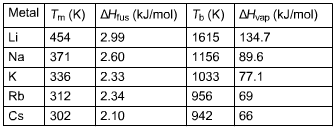# Problem: Using the following data,Calculate ?Sfus and ?Svap for Cs.

###### FREE Expert Solution

To calculate ΔSfus and ΔSvap for Cs:

$\overline{){{\mathbf{\Delta S}}}_{{\mathbf{fus}}}{\mathbf{=}}\frac{{\mathbf{\Delta H}}_{\mathbf{fus}}}{{\mathbf{T}}_{\mathbf{m}}}}$ $\overline{){{\mathbf{\Delta S}}}_{{\mathbf{vap}}}{\mathbf{=}}\frac{{\mathbf{\Delta H}}_{\mathbf{vap}}}{{\mathbf{T}}_{\mathbf{b}}}}$###### Problem Details

Using the following data,Calculate ?Sfus and ?Svap for Cs.